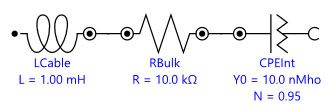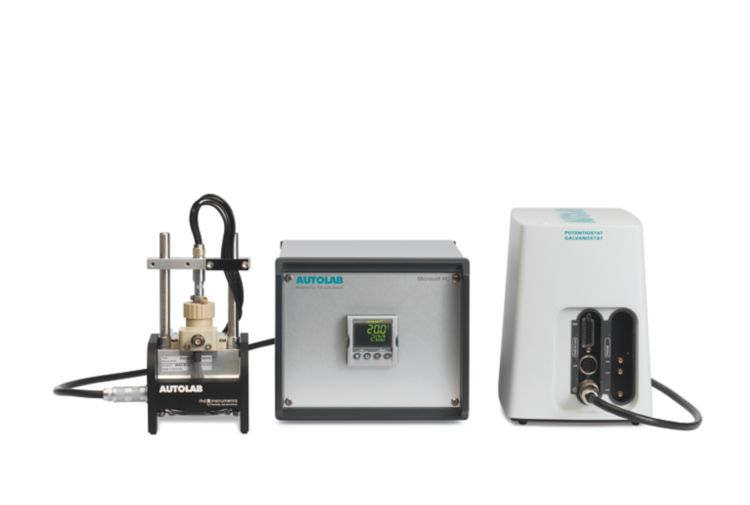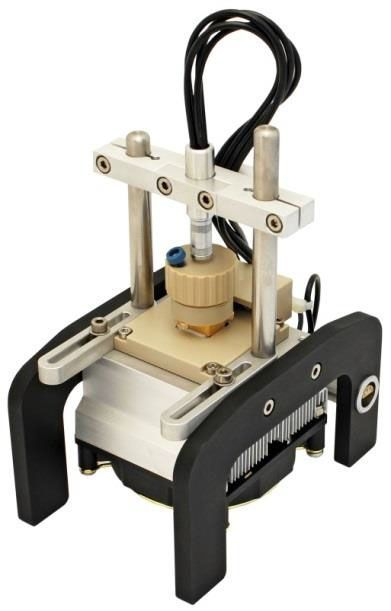You have been redirected to your local version of the requested page

One approach for improving the performance of energy storage devices (e.g., batteries and supercapacitors) is to enhance the ion conductivity (𝜎𝐷𝐶, 𝑆 ⋅ 𝑐𝑚-1) of the electrolyte. The common method to obtain 𝜎𝐷𝐶 is to perform electrochemical impedance spectroscopy (EIS) experiment at different temperatures using a 2-electrode setup. For routine measurements of a large number of samples, fast exchange of sample compartments or if an automatic sample analysis is desired this approach is very convenient as it reduces errors and saves time. Metrohm Autolab provides a measurement setup, the Autolab Microcell HC, which can be combined with an Autolab instrument with a FRA32M module allowing for an automatic determination of temperature dependent 𝜎𝐷𝐶 values. In this application note, general information about the basics of 𝜎𝐷𝐶 determination as well as an exemplary study of 𝜎𝐷𝐶(𝑇) for a typical Li-ion battery electrolyte are presented.Figure 1. Equivalent circuit describing the interface between a good ion conductor and an inert electrode

When a good liquid ion conductor is in contact with a blocking electrode, the recorded EIS data of most real systems can be described by a serial connection of an inductor (LCable) representing the inductance of the cables connecting the electrodes with the instrument, an ohmic resistor (RBulk) describing the resistance for bulk ion transport, and a constant phase element (CPEInt), which takes into account a non-ideal capacitive behaviour of the interface. (see Figure 1).

It is a common procedure to analyse EIS data in the Nyquist plot. The equivalent circuit shown in Figure 1 leads to a slightly curved line due to the non-ideal capacitive behaviour of the interface, which intersects the Z' axis at RBulk, at high frequencies (see Figure 2).

Figure 2. Nyquist plot corresponding to the equivalent circuit shown in Figure 1.

However, the Nyquist plot is not the best data representation for a proper analysis, because in a high frequency regime artefacts, either due to the cable’s impedance or the presence of bulk impedance, lead to a second intercept with the real axis of the Nyquist plot. Therefore, care has to be taken to avoid misinterpretations concerning the RBulk value.

Instead of the Nyquist plot, a Bode plot of the modulus of the admittance, Y (in Siemens, S or Mho) should be preferred. The relationship between the impedance Z and the admittance are given by:

Where the real (Y') and imaginary (Y'') parts of the admittance are given by:

The Bode plot of the admittance modulus Y for the equivalent circuit of Figure 1 is shown in Figure 3.

Figure 3. Bode plot of 𝑌 for the equivalent circuit shown in Figure 1

At high frequencies, the admittance values decrease with increasing frequency. This is caused by the inductance of the cables. At lower frequencies the curve is parallel to the frequency axis. This part is governed by bulk ion transport and the value of the admittance is identical to 𝜎DC /KCell . Here, KCell (𝑐𝑚−1) is the cell constant which can be calculated via an EIS measurement of an applicable conductivity standard, such as the Metrohm conductivity standard 100 𝜇𝑆/𝑐𝑚.

At lower frequencies, the charging of the interfacial capacitance is observable which causes a decrease of the admittance values.

After fitting the recorded data to the equivalent circuit in Figure 1, the reciprocal of the obtained value for RBulk can be multiplied with the cell constant KCell to calculate 𝜎DC:

However, there are additional experimental considerations. First of all 𝜎DC shows a significant temperature dependence which can often be described by an empirical Vogel-Fulcher-Tamman approach:

With 𝜎0, A and Tg the fitting parameters. Therefore, the sample temperature has to be controlled. Often, the temperature of the sample compartment is controlled via an external circulating bath, which is a relatively time-consuming procedure.

Secondly, most modern electrolytes are volatile and require leak-tight sample compartments, which allow for measurements within a broad temperature range.

Finally, although the EIS experiments are fast since only the high frequency impedance has to be recorded, the data analysis might be time consuming. An analysis tool for the measured EIS data like the Fit and Simulation Command found in NOVA is highly welcome.Figure 4. The Autolab Microcell HC combined with the Autolab PGSTAT204 and the FRA32M module

The combination of the measurement setup Autolab Microcell HC with a Metrohm Autolab potentiostat/galvanostat instrument equipped with a FRA32M module, as shown in Figure 4, provides a temperature-controlled electrochemical measurement system for volatile samples.Figure 5. Overview of the cell holder and the electrochemical cell

The cell is fitted with a glass-sealed platinum wire working electrode and a platinum crucible counter electrode. The cell is then connected to a cell holder capable to control the temperature of the cell via a Peltier element, see Figure 5.

The cell holder is connected to the temperature controller, itself connected to the PC via a serial RS-232 interface, allowing for an automated temperature control.

Through the dedicated NOVA commands, the Autolab Microcell HC offers the following unique advantages:

• Possibility to define a temperature range (in this application note: from 5 °C to 60 °C).
• Possibility to define stability conditions (in this application note 0.5 °C/min) as well as waiting time for maximum temperature deviation.
• Possibility to define a hold time after fulfilling the stability conditions.

For the measurements presented in this application note, the measurement cell is filled with 1.0 mL of 1 M LiClO4 solution in ethylene carbonate/dimethyl carbonate 1:1. For the determination of the 𝐾𝐶𝑒𝑙𝑙 value, the Metrohm conductivity standard 100 𝜇𝑆/𝑐𝑚 (6.2324.010) has been used.

The impedance is sampled at open circuit potential within a frequency range from 250 kHz to 1 kHz applying an AC amplitude of 10 mV (RMS). Using the Fit and Simulation Command in NOVA, the recorded data are subjected to a fitting procedure using a serial LRQ equivalent circuit i, as shown in Figure 1. Impedance spectra are measured for temperatures ranging from 5 °C to 60 °C in steps of 5 °C.

The measurement temperatures as well as the temperature stability values can also be specified. A message box allows the value of the cell constant KCell to be specified. In this application note, KCell is set to 15.6 cm-1.

After inserting the KCell  value, the EIS measurement of the sample within the chosen temperature range is carried out.

Once the measurements starts, NOVA shows the impedance Nyquist plot, the Bode plot of the impedance modulus Z and phase 𝜑; the Bode plots of the admittance modulus Y, the plots of the time dependence of the AC-current and AC-voltage and the Lissajous plots, per frequency.

When the measurement is finished, the Arrhenius plot of the 𝜎DC conductivity is shown, Figure 6.

Figure 6. The Arrhenius plot of the 𝜎𝐷𝐶 conductivity.

According to Figure 6, the natural logarithm of the conductivity at 25 °C (3.35 1000/K) is ≈ 4.8, resulting in a conductivity of 8.2 mS/cm, in accordance with literature data, 8.4 mS/cm.

The combination of the Autolab Microcell HC setup with the Metrohm Autolab potentiostat/galvanostat instruments fitted with the FRA32M module allows for an automatic determination of the temperature-dependent 𝜎DC values. This convenient combination offers the possibility to significantly reduce the time spent on performing measurements and analyzing the recorded data.

1. Kang Xu, “Nonaqueous Liquid Electrolytes for Lithium-Based Rechargeable Batteries”, Chemical Reviews, 2004, Vol. 104, No. 10.